# Qlik Design Blog

All about product and Qlik solutions: scripting, data modeling, visual design, extensions, best practices, etc.

Announcements
QlikWorld Online 2021, May 10-12: Our Free, Virtual, Global Event REGISTER TODAYEmployee

## Aggregations and Function Classes

A typical QlikView application may have one million records in the data, one hundred rows in a pivot table and a single number, a KPI, in a gauge or text box. Although different in magnitudes, all three numbers may still represent all data. The numbers are just different aggregation levels.

There are many functions in QlikView that can help you write the necessary formulae to calculate aggregated KPI:s. Some will collapse many records into one value, others will not. Today I will write about the different function classes, and how you can combine them.

•The Scalar Functions constitute the first class. Typical for these is that they are one-to-one functions, i.e. they take a single value as parameter and return a single value (of the dual data type). Examples: Left(), If(), Num(), Date(), Year(), Subfield(), etc.
•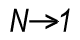The Aggregation Functions constitute the second class. These are many-to-one functions, i.e. they use the values from many records as input and collapse these into one single value that summarizes all records.  Examples: Sum(), Count(), Avg(), Min(), Only(), Concat(), etc.

Aggregation functions are special: You must use one to collapse several records into one number – which means that you need them in pretty much any formula in QlikView: In Chart expressions, in Text boxes, in Labels, etc. If you don’t write an aggregation function in your expression, QlikView will assign one for you: It will use the Only() function.

Scalar functions can be used both inside and outside the aggregation function: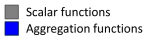Date( Min( Date )

Money( Sum( If( Group='A', Amount ) ) )

There is one restriction: You can normally not use an aggregation function inside another aggregation function. Hence, you usually need every field reference to be wrapped in exactly one aggregation function.

•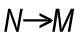The next function class has only one member: The Aggr Function. It is – in spite of its name – not an aggregation function. It is a many-to-many function, rather like a tensor or a matrix in mathematics. It converts a table with N records to a table with M records. In other words: It returns an array of values. Regard it as a virtual straight table with one measure and one or several dimensions.

Most places in QlikView demand that you write your expression so that it returns one single value. This means that you must wrap the Aggr function in an aggregation function to get a meaningful result. The only exception is if you use the Aggr function to define a calculated dimension or field. This means that you have two aggregation steps; one nested in the other:Avg( Aggr( Sum( Amount ), Month ) )

Charts complicate the matters slightly: A chart is like a For-Next loop where the number of distinct dimension values determines the number of loops. In each loop, the expression must return one value only, and this is the value used for the bar/slice/pivot table row.

However, sometimes you need values from other rows in the chart, and it could even be that you need values from several rows. To solve this, there are two additional classes of functions that should be used together:

•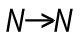The Chart Inter-record Functions return values fetched from other rows in the chart. Some of these can return several values, i.e. an array of values. These functions are only meaningful inside a chart or Aggr() function. Examples: Above(), Below(), Top(), etc.
•The Range Functions are functions that can collapse a chart inter-record array into one single value. Examples: RangeSum(), RangeMin(), RangeMax(), etc.

Example: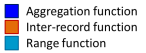RangeSum( Above( Sum( Amount ), 0, 12 ) )

Bottom line: Know your functions. It will help you write correct expressions.

HIC

See also

It’s all Aggregations

Dimensions and Measures

The Above Function

31 Comments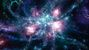Partner

Hi HIC,

Thank you for your great blog posts. To fix all the confusion, why isn't the Aggr() function called something else like Multi() to prevent mixing with other Aggregation functions Sum, Min, Max?

Paul

3,640 ViewsEmployee

I agree that the name isn't optimal... But at this stage it would probably cause even more confusion if we renamed the function.

HIC

3,640 ViewsNot applicable

Hi Henric Great Blog

Can you please expalin us how to compare the top n values in selected month to the same values in previous month

this is one of the problems we always face

3,640 ViewsEmployee

I would probably do something like the following:

Create at pivot table with Month as first dimension, and

=Aggr(If(Rank(Sum(Sales),4,1)<=5,Rank(Sum(Sales),4,1)),YearMonth,Customer)

as second dimension called "Rank".

With this you will get the top 5 Customers per month. If you use Only(Customer) as measure, you will getbut you can of course use other measures, e.g.

Sum(Sales)- Above(Sum(Sales))

which should be pretty close to what you want.

HIC

3,640 ViewsNot applicable

Hi Henric

Thank you for the reply

The asof calender by you, solved my entire problem prviously and that one calender will solve all the rolling issues. that is brilliant way and easy way to solve problems.

The rank function is providing me good results in other senario.

But

My question is actually when we select a month the top 3 values in that month will be displayed

and can we see how these top 3 values in current month are in previous month(comparitive analysis kind of)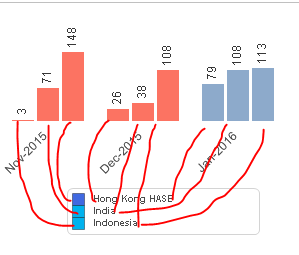i am bit worried if we can do it in qlikview

your answer would be greatly helpfull

3,640 ViewsContributor III

‌You can use the P() function in your set analysis.

CompanyID = P({<Aggr(If(Rank(Sum(Sales),4,1)<=5,Rank(Sum(Sales),4,1)),YearMonth,Customer)  >} CompanyID)

You might have to play with the P() but it should give you desired result.

3,640 Views